1．设f (x)x0处可导，g (x)x0处不可导，那么在x0           .

Af (x)+ g (x) f (x)g (x)x0处都不可导；

Bf (x)+ g (x) f (x)g (x)x0处都可导；

Cf (x)+ g (x) 未必不可导，而 f (x)g (x)一定不可导；

Df (x)+ g (x) 一定不可导，而 f (x)g (x)未必不可导；

2．设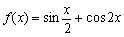，则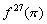的值等于           .

A0 B）－227  C227227  D227

3．设f (x) [a, b]上连续，积分中值定理：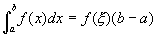中的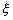.

A[a, b]上任一点；            B）在[a, b]上至少存在某一点；

C[a, b]上唯一的某一点；      D[a, b]上的中点

4．设函数y = f (x)可导，且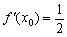，则当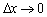时，该函数在x0处的微分是       .

A）Δx的等阶无穷小         B）Δx的同阶无穷小

C）Δx的高阶无穷小；         D）Δx的低阶无穷小

1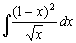=           .

2．函数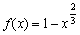[-11]上不能有罗尔定理的结论，其原因是由于f (x)不满足罗尔定理           的条件。

3．设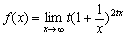，则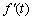4．设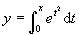，则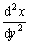=          .

5．由曲线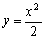和直线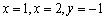所围成的图形绕直线y=1旋转一周所得旋转体的体积可用定积分表示为          .

三、计算题（4×3=52分）

1．求极限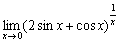2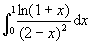3．求曲线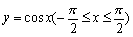x轴所围图形绕x轴旋转一周的旋转体之体积。

4．求极限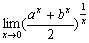. 5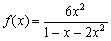，求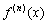.

6．求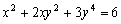x=1处的切线方程.

7．设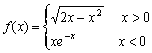，求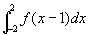8．设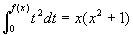，且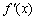存在，求f (2).

9．已知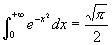，求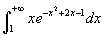.

10．求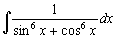.

五、设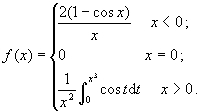讨论f (x)x = 0处的连续性和可导性。（5分）

①对称轴平行于y轴，且图形是的。

②它与x轴所围成的面积最小。（5分）